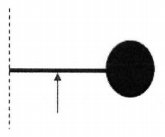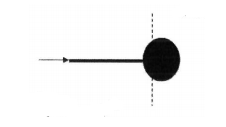# Problem:  An object is formed by attaching a uniform, thin rod with a mass of mr = 6.85 kg and length L = 5.76 m   to a uniform sphere with mass ms = 34.25 kg and radius R = 1.44 m.  Note ms = 5mr and L = 4R.1) What is the moment of inertia of the object about an axis at the left end of the rod?  kg-m2  2) If the object is fixed at the left end of the rod, what is the angular acceleration if a force F = 460 N is exerted perpendicular to the rod at the center of the rod?rad/s2  3) What is the moment of inertia of the object about an axis at the center of mass of the object?  (Note: the center of mass can be calculated to be located at a point halfway between the center of the sphere and the left edge of the sphere.)  kg-m2  4) If the object is fixed at the center of mass, what is the angular acceleration if a force F = 460 N  is exerted   parallel to the rod at the end of rod?rad/s2  5)  What is the moment of inertia of the object about an axis at the right edge of the sphere?

###### FREE Expert Solution

Moment of inertia for a point mass:

$\overline{){\mathbf{I}}{\mathbf{=}}{\mathbf{M}}{{\mathbf{R}}}^{{\mathbf{2}}}}$

Moment of inertia of a solid sphere:

$\overline{){\mathbf{I}}{\mathbf{=}}\frac{\mathbf{2}}{\mathbf{5}}{\mathbf{M}}{{\mathbf{R}}}^{{\mathbf{2}}}}$

Moment of inertia of a rod about the end:

$\overline{){\mathbf{I}}{\mathbf{=}}\frac{\mathbf{1}}{\mathbf{3}}{\mathbf{M}}{{\mathbf{L}}}^{{\mathbf{2}}}}$

Newton's second law for the angular motion:

$\overline{){\mathbf{\tau }}{\mathbf{=}}{\mathbf{I}}{\mathbf{\alpha }}}$, where τ is the net external torque, I is the moment of inertia, and α is the angular acceleration.

Torque:

$\overline{){\mathbf{\tau }}{\mathbf{=}}{\mathbf{r}}{\mathbf{×}}{\mathbf{F}}}$

91% (70 ratings)###### Problem Details

An object is formed by attaching a uniform, thin rod with a mass of mr = 6.85 kg and length L = 5.76 m   to a uniform sphere with mass ms = 34.25 kg and radius R = 1.44 m.  Note ms = 5mr and L = 4R.1) What is the moment of inertia of the object about an axis at the left end of the rod?

kg-m2

2) If the object is fixed at the left end of the rod, what is the angular acceleration if a force F = 460 N is exerted perpendicular to the rod at the center of the rod?

rad/s2

3) What is the moment of inertia of the object about an axis at the center of mass of the object?  (Note: the center of mass can be calculated to be located at a point halfway between the center of the sphere and the left edge of the sphere.)kg-m2

4) If the object is fixed at the center of mass, what is the angular acceleration if a force F = 460 N  is exerted   parallel to the rod at the end of rod?

rad/s2

5)  What is the moment of inertia of the object about an axis at the right edge of the sphere?

Frequently Asked Questions

What scientific concept do you need to know in order to solve this problem?

Our tutors have indicated that to solve this problem you will need to apply the Moment of Inertia & Mass Distribution concept. You can view video lessons to learn Moment of Inertia & Mass Distribution. Or if you need more Moment of Inertia & Mass Distribution practice, you can also practice Moment of Inertia & Mass Distribution practice problems.